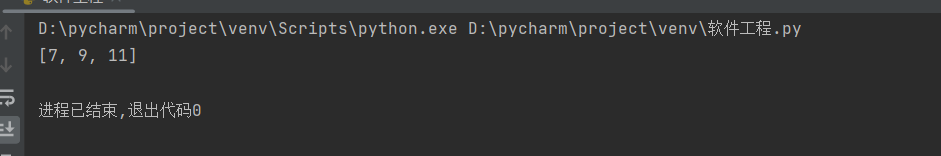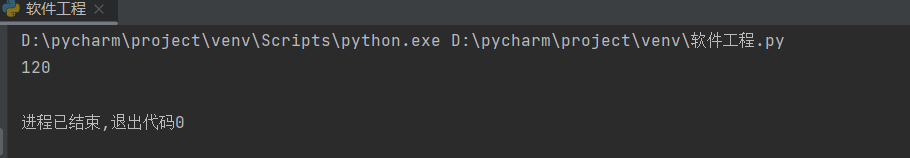# 2023（春）Python程序设计作业3： 函数及应用

## 一 、函数定义及使用

### 例如

``````def calculate(x, y):
if y == 0:
return None
else:
return x + y, x - y, x * y, round(x / y, 2)

x, y = 5, 3
print(calculate(x, y))``````

## 二、Lambda函数

### 例如

``````numbers = [1, 3, 5, 7, 9, 11]
n = 5
greater_than_n = list(filter(lambda x: x > n, numbers))
print(greater_than_n)``````## 三、递归函数

### 例如

``````def factorial(n):
if n == 1:
return 1
else:
return n * factorial(n - 1)

n = 5
print(factorial(n))``````...全文
11 回复 打赏 收藏 转发到动态 举报2023（Python程序设计作业5：Pandas基础技能及综合应用
【代码】2023（Python程序设计作业5：Pandas基础技能及综合应用2023（Python程序设计作业6：基于unitest的Python测试
【代码】2023（Python程序设计作业6：基于unitest的Python测试。2023（Python程序设计作业1： 基础语法之控制流程2023（Python程序设计作业4：Numpy 和 Pillow在图像处理中的应用大工18《c和c语言程序设计》,东大21《高级语言程序设计基础》在线平时作业1题目及答案...

162发帖与我相关我的任务
python 高校 江苏省·南通市• 近7日
• 近30日
• 至今